## MTSO July News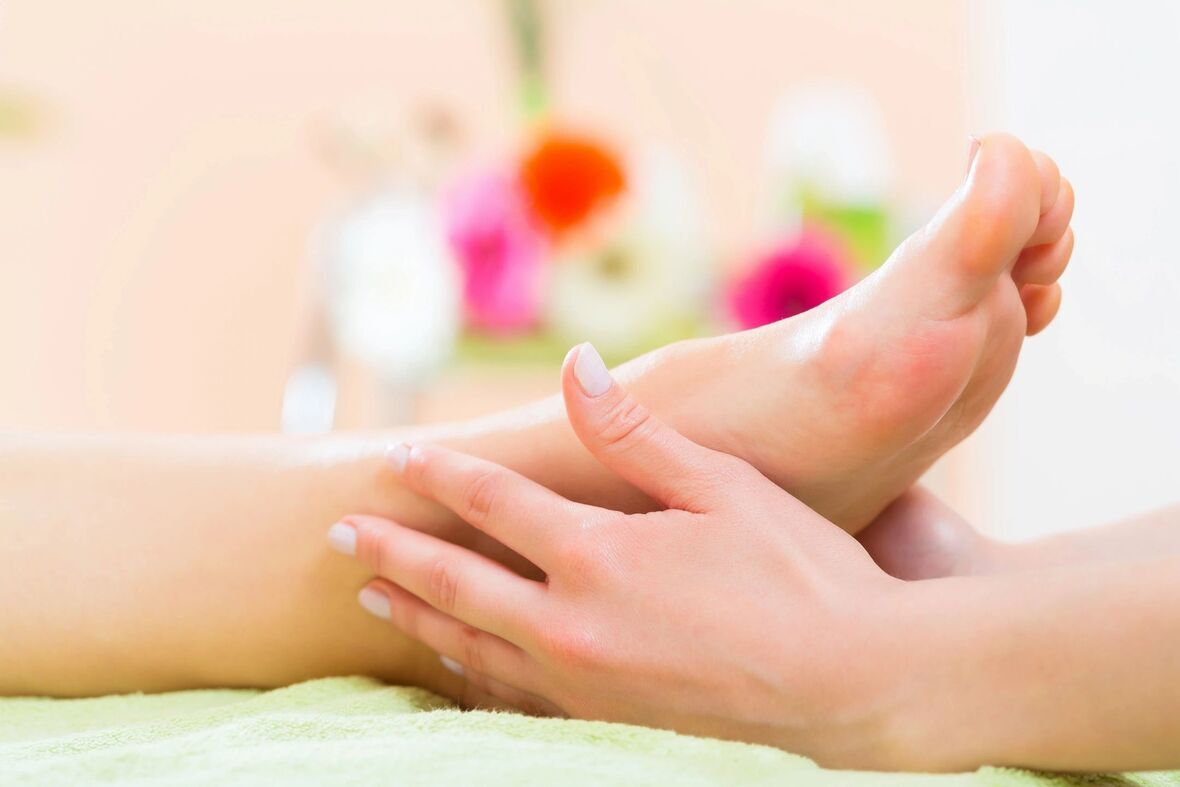July brings flowers blooming, gardens growing, and easy enjoyment of the great outdoors. We hope you are taking the time to enjoy the sunshine and remembering to practice self-care. MTSO is here to help you and supply you with your needs. Our knowledgeable staff is more than happy to assist you with questions you may have.

Looking for upgrades to your clinic or home office? Call us at 780-440-1818 for suggestions on how to update your workspace with an affordable electric table, a sweet aromatherapy program, or new sheets.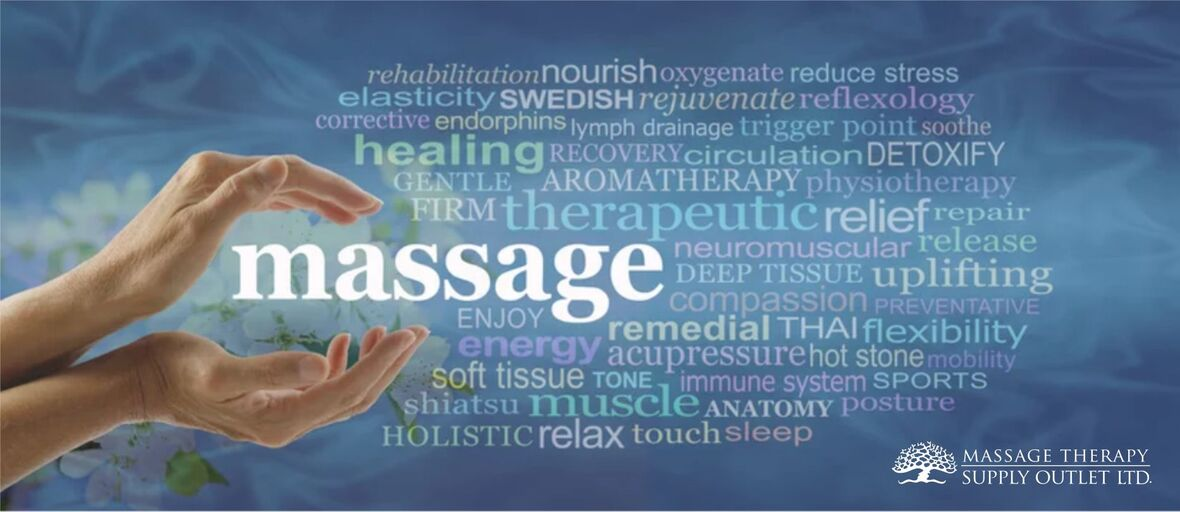table div table+table+table+table+table div table{width:100%;padding:0}table div table+table+table+table+table div table img{width:96.23%;padding:0;float:none}table div table+table+table+table+table div table td{width:100%;padding:0 1.88% 18px}/* styles *//* styles */

# MARBLE-LOOK SHEET SET

\$42.95
The marble look of these lovely cotton sheet sets minimizes any oil stains! Includes top sheet, fitted sheet, face cradle cover. (Note: coloured on one side only)

100% cotton flannel with flat sheet size of 52.5" x 94" and fitted sheet that fits up to a 29" wide table

Locally crafted to RMT specifications. We are proud to ship our locally-produced linens throughout Canada.

 table div table+table+table+table+table+table+table+table+table div table{width:100%;padding:0}table div table+table+table+table+table+table+table+table+table div table img{width:96.23%;padding:0;float:none}table div table+table+table+table+table+table+table+table+table div table td{width:100%;padding:0 1.88% 18px}/* styles */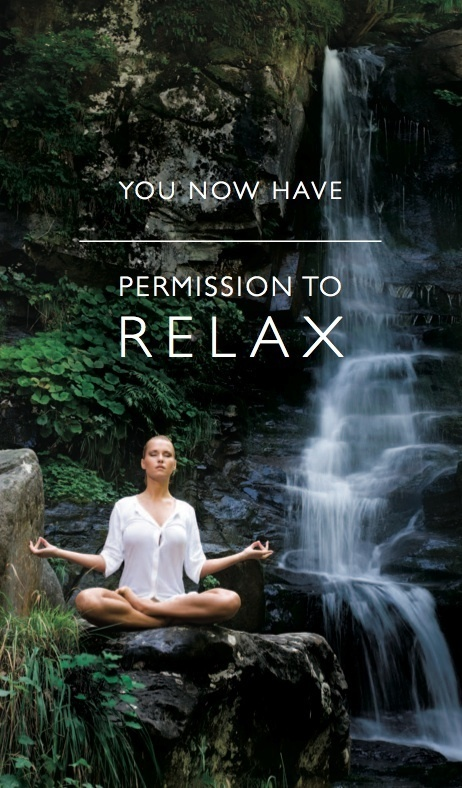# Here's the perfect natural bug spray recipe from Marie, our resident aromatherapist.

* LAVENDER
* EUCALYPTUS
* LEMONGRASS
* GERANIUM
* CINNAMON LEAF
* PEPPERMINTClick on image to enlarge Recipe
 table div table+table+table+table+table+table+table+table+table+table+table+table+table div table{width:100%;padding:0}table div table+table+table+table+table+table+table+table+table+table+table+table+table div table img{width:96.23%;padding:0;float:none}table div table+table+table+table+table+table+table+table+table+table+table+table+table div table td{width:100%;padding:0 1.88% 18px}/* styles */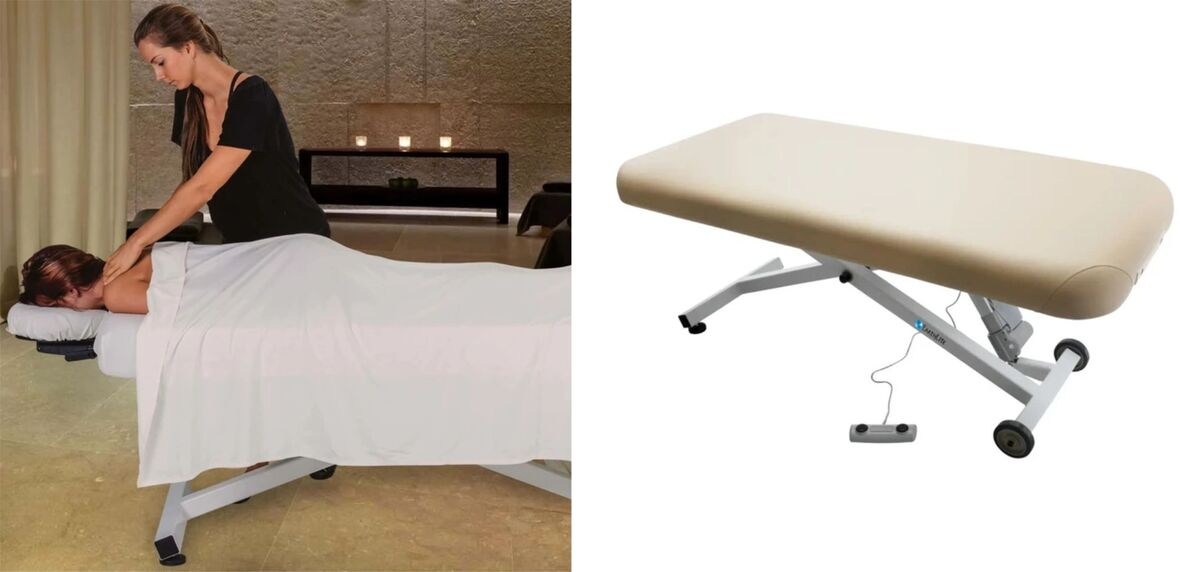STRONGLITE ERGO FLAT TOP ELECTRIC TABLE

# STRONGLITE ERGO FLAT TOP ELECTRIC TABLE

The quietest, most popular spa hydraulic lift massage table. Made in USA. Our Ergo™ electric lift table is designed for comfort, long-lasting reliability, easy care, and great performance making this table a superb value.

\$1,799.00
* Smooth, reliable electric lift actuator
* Rounded Corners
* Heavy Duty Steel Frame
* Natursoft™ Upholstery – 100% PU
* Pro-Plush™ Deluxe 3-Layer 3” Cushioning System
* Wheels For Ease Of Movement
* Easy to use foot pedal

 table div table+table+table+table+table+table+table+table+table+table+table+table+table+table+table+table+table div table{width:100%;padding:0}table div table+table+table+table+table+table+table+table+table+table+table+table+table+table+table+table+table div table img{width:96.23%;padding:0;float:none}table div table+table+table+table+table+table+table+table+table+table+table+table+table+table+table+table+table div table td{width:100%;padding:0 1.88% 18px}/* styles */# LIQUID SUNSHINE™ MASSAGE OIL

An ideal natural blend of 3 oils providing nourishing skin care for northern climates. This nut-free, unscented oil is suitable for a wide variety of massage applications.

▪ Sunflower Seed oil, high in vitamins A, B, D & E, minerals & unsaturated fatty acids, is light and easily absorbed.
▪ Fractionated Coconut oil, also high in vitamin E, is a smooth, emollient, easily absorbed oil, providing consistent glide and excellent transport for Aromatherapy oils.
▪ Jojoba oil being rich in vitamins A, D & E, is also soothing and emollient.
▪ The effect is that both client and therapist finish with smooth moisturized skin.
▪ The inclusion of a natural water dispersant assures easy laundering.
 ▪ Sunflower Seed oil, high in vitamins A, B, D & E, minerals & unsaturated fatty acids, is light and easily absorbed.
 ▪ Fractionated Coconut oil, also high in vitamin E, is a smooth, emollient, easily absorbed oil, providing consistent glide and excellent transport for Aromatherapy oils.
 ▪ Jojoba oil being rich in vitamins A, D & E, is also soothing and emollient.
 ▪ The effect is that both client and therapist finish with smooth moisturized skin.
 ▪ The inclusion of a natural water dispersant assures easy laundering.

Available in 2 sizes: 1 litre & 4 litres

 table div table+table+table+table+table+table+table+table+table+table+table+table+table+table+table+table+table+table+table+table div table{width:100%;padding:0}table div table+table+table+table+table+table+table+table+table+table+table+table+table+table+table+table+table+table+table+table div table img{width:96.23%;padding:0;float:none}table div table+table+table+table+table+table+table+table+table+table+table+table+table+table+table+table+table+table+table+table div table td{width:100%;padding:0 1.88% 18px}/* styles */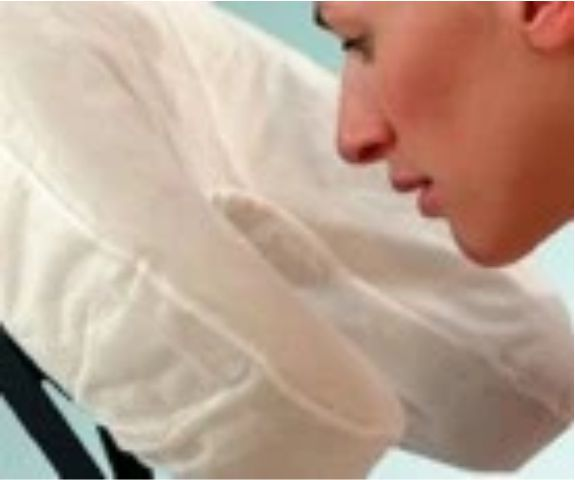Fitted Disposable Face Cradle Covers

# FITTED DISPOSABLE FACE CRADLE COVERS

Made from soft, 25 gsm spunbound fabric, this new and innovative design provides a comfortable, sanitary and disposable barrier for your clients and an easy changeover for between client protection.

▪ Fits all crescent-shaped face rests
▪ Stays put throughout your treatment
▪ Great for table and seated massage
▪ Box of 50
▪ Latex Free

 table div table+table+table+table+table+table+table+table+table+table+table+table+table+table+table+table+table+table+table+table+table+table+table div table{width:100%;padding:0}table div table+table+table+table+table+table+table+table+table+table+table+table+table+table+table+table+table+table+table+table+table+table+table div table img{width:96.23%;padding:0;float:none}table div table+table+table+table+table+table+table+table+table+table+table+table+table+table+table+table+table+table+table+table+table+table+table div table td{width:100%;padding:0 1.88% 18px}/* styles */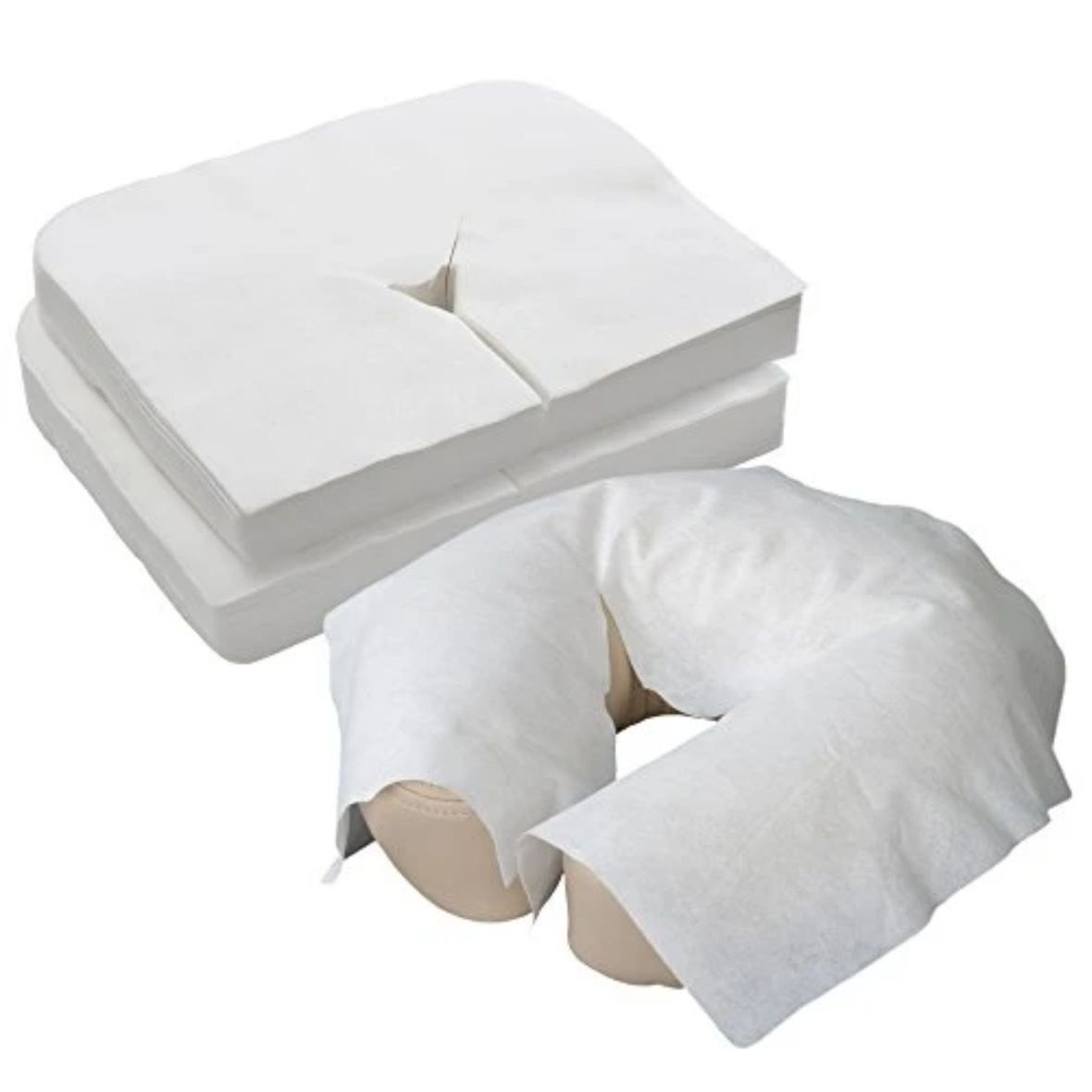Flat Disposable Face Cradle Covers

# FLAT DISPOSABLE FACE CRADLE COVERS

Flat soft disposable face cradle covers package of 100. Product stores easily in table and chair carry cases. Thick, yet soft and silky fabric conforms to the shape of your cushion. Feels like napped cotton. Flat face covers fit almost anything.

 table div table+table+table+table+table+table+table+table+table+table+table+table+table+table+table+table+table+table+table+table+table+table+table+table+table+table div table{width:100%;padding:0}table div table+table+table+table+table+table+table+table+table+table+table+table+table+table+table+table+table+table+table+table+table+table+table+table+table+table div table img{width:96.23%;padding:0;float:none}table div table+table+table+table+table+table+table+table+table+table+table+table+table+table+table+table+table+table+table+table+table+table+table+table+table+table div table td{width:100%;padding:0 1.88% 18px}/* styles */table.module-26{width:95.85%;padding:0}table div table+table+table+table+table+table+table+table+table+table+table+table+table+table+table+table+table+table+table+table+table+table+table+table+table+table+table div table{width:95.85%;float:none;margin-left:auto;margin-right:auto;padding:0}table div table+table+table+table+table+table+table+table+table+table+table+table+table+table+table+table+table+table+table+table+table+table+table+table+table+table+table div table a{border:0 none;text-decoration:none}table div table+table+table+table+table+table+table+table+table+table+table+table+table+table+table+table+table+table+table+table+table+table+table+table+table+table+table div table img{width:100%!important;border:0 none;text-decoration:none}table div table+table+table+table+table+table+table+table+table+table+table+table+table+table+table+table+table+table+table+table+table+table+table+table+table+table+table div table td{width:100%;padding:0}/* styles */
 table div table+table+table+table+table+table+table+table+table+table+table+table+table+table+table+table+table+table+table+table+table+table+table+table+table+table+table+table+table div table{width:100%;padding:0}table div table+table+table+table+table+table+table+table+table+table+table+table+table+table+table+table+table+table+table+table+table+table+table+table+table+table+table+table+table div table img{width:96.23%;padding:0;float:none}table div table+table+table+table+table+table+table+table+table+table+table+table+table+table+table+table+table+table+table+table+table+table+table+table+table+table+table+table+table div table td{width:100%;padding:0 1.88% 18px}/* styles */# MTSO SUMMER HOURS

From July 1 - August 15, 2021, we will be open

Monday - Friday from 10 am - 5 pm and on Saturdays from 10 am - 4-pm

Our online store is always open: www.mtso.ab.ca

 table div table+table+table+table+table+table+table+table+table+table+table+table+table+table+table+table+table+table+table+table+table+table+table+table+table+table+table+table+table+table+table+table div table{width:100%;padding:0}table div table+table+table+table+table+table+table+table+table+table+table+table+table+table+table+table+table+table+table+table+table+table+table+table+table+table+table+table+table+table+table+table div table img{width:96.23%;padding:0;float:none}table div table+table+table+table+table+table+table+table+table+table+table+table+table+table+table+table+table+table+table+table+table+table+table+table+table+table+table+table+table+table+table+table div table td{width:100%;padding:0 1.88% 18px}/* styles */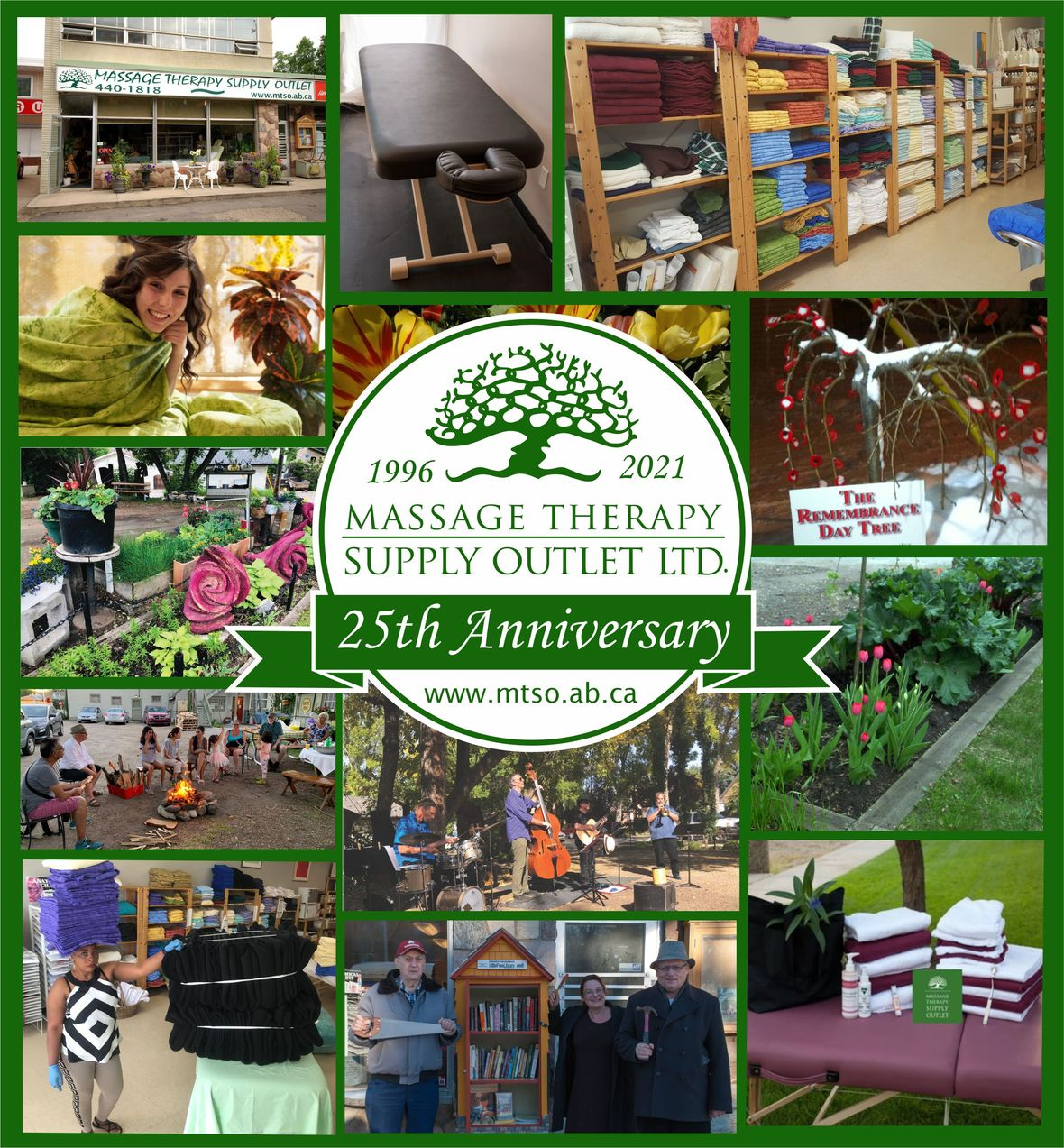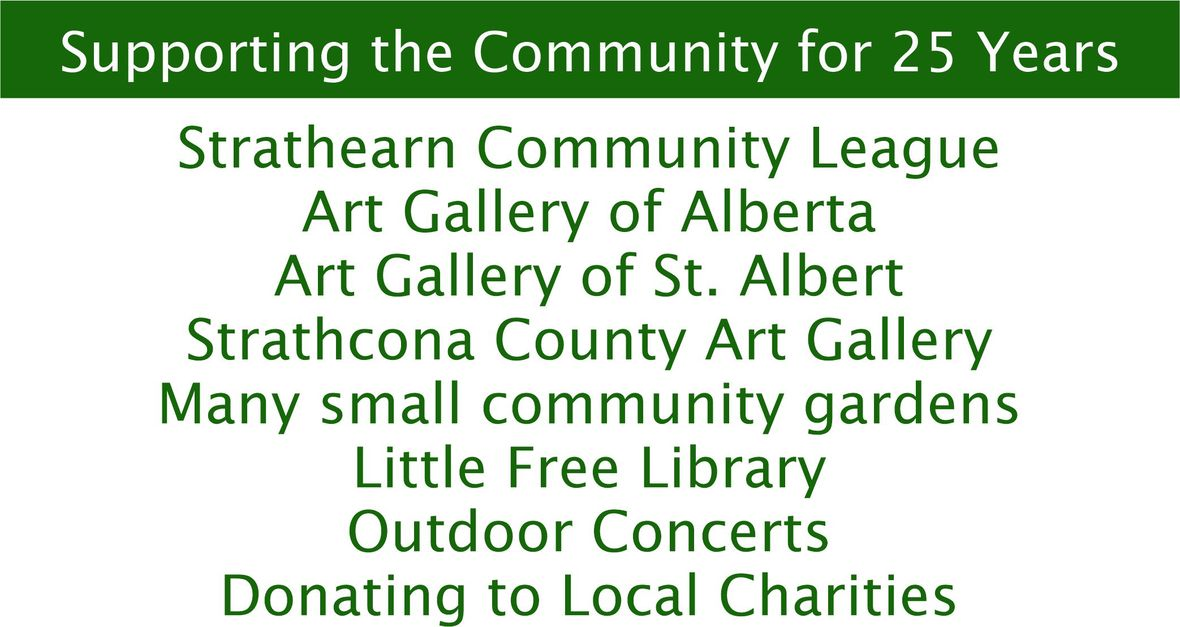table div table+table+table+table+table+table+table+table+table+table+table+table+table+table+table+table+table+table+table+table+table+table+table+table+table+table+table+table+table+table+table+table+table+table+table+table div table{width:100%;padding:0}table div table+table+table+table+table+table+table+table+table+table+table+table+table+table+table+table+table+table+table+table+table+table+table+table+table+table+table+table+table+table+table+table+table+table+table+table div table img{width:96.23%;padding:0;float:none}table div table+table+table+table+table+table+table+table+table+table+table+table+table+table+table+table+table+table+table+table+table+table+table+table+table+table+table+table+table+table+table+table+table+table+table+table div table td{width:100%;padding:0 1.88% 18px}/* styles */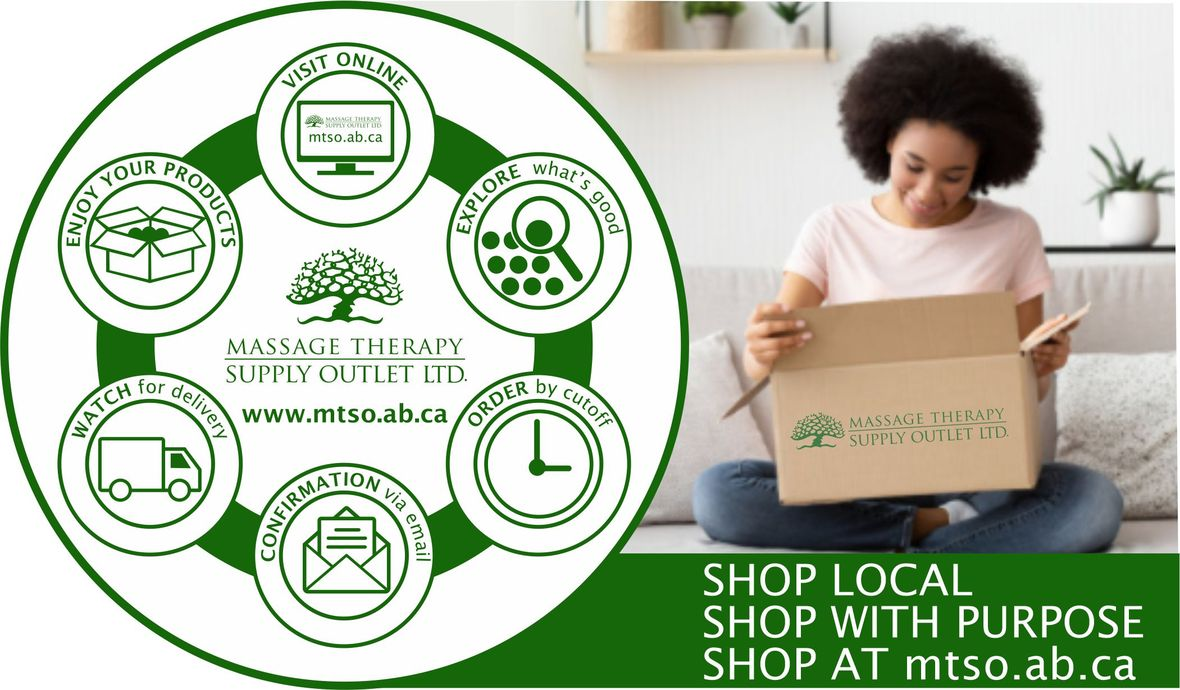# CURBSIDE PICKUP IS AVAILABLE

In addition to in-store shopping and shipping, curbside pick-up is also available. You can either phone-in your order or place order online. When placing your order online choose Local Pick Up at check out. We will contact you when your order is ready.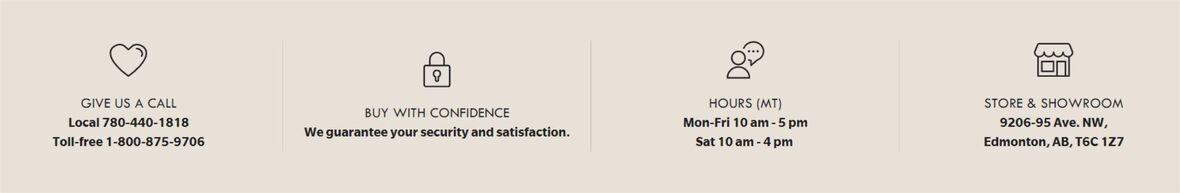/* styles */ Browse and buy from the extensive collection of quality products at our online store, always open, 24/7/365. Drop by our Edmonton store and showroom: 10:00 - 5:00 Mon-Fri, 10:00-4:00 Sat.
 Like   Tweet   Pin   in